# Formula of metacentric height

• foo9008

## Homework Statement

is I/ V ( in the second picture)submerged is a valid formula of metacentric height ? i have never seen this in my textbook . This is just another notes given by my senior.

## Homework Statement

is I/ V ( in the second picture)submerged is a valid formula of metacentric height ? i have never seen this in my textbook . This is just another notes given by my senior.

## The Attempt at a Solution

It seems to agree with https://en.wikipedia.org/wiki/Metacentric_height

which part ? i didnt see it
At https://en.wikipedia.org/wiki/Metacentric_height#Metacentre it gives the formula BM=I/V

Edit: After SteamKing's post I realized I had misread the Wikipedia text. SteamKing is right, the metacentric height is from the centre of gravity to the metacentre, whereas I/V is from the centre of buoyancy to the metacentre.
Sorry for the confusion.

Last edited:
•foo9008 and gracy

## Homework Statement

is I/ V ( in the second picture)submerged is a valid formula of metacentric height ? i have never seen this in my textbook . This is just another notes given by my senior.

## The Attempt at a Solution

I / V gives the distance between the metacenter M and the center of buoyancy B. In other words, BM = I / V.

The metacentric height of a vessel is usually taken as the distance between the center of gravity G and the metacenter and is referred to as the GM. A stable vessel will have a positive value of GM, i.e., G is always located below M.

The calculation of the distance GM is usually obtained from the following formula:

GM = KB + BM - KG

where K denotes the location of the bottom of the vessel, making KB the vertical location of the center of buoyancy, KG the vertical location of the center of gravity, and BM the location of the metacenter from the center of buoyancy.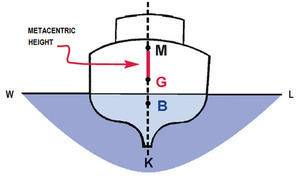•foo9008, gracy and haruspex
I / V gives the distance between the metacenter M and the center of buoyancy B. In other words, BM = I / V.

The metacentric height of a vessel is usually taken as the distance between the center of gravity G and the metacenter and is referred to as the GM. A stable vessel will have a positive value of GM, i.e., G is always located below M.

The calculation of the distance GM is usually obtained from the following formula:

GM = KB + BM - KG

where K denotes the location of the bottom of the vessel, making KB the vertical location of the center of buoyancy, KG the vertical location of the center of gravity, and BM the location of the metacenter from the center of buoyancy.metacenter is the distance between G and M , right ? why the author gave the MB is 0.03m away from center of buoyancy ? why M is not 0.03 m away from G ?

metacenter is the distance between G and M , right ? why the author gave the MB is 0.03m away from center of buoyancy ? why M is not 0.03 m away from G ?
The author did not just pull the distance 0.03 m out of the air. BM is a calculated value. That's what BM = I / V means, and that's why it gives the distance between B and M and not G and M.

The distance BM is a function of the inertia of the waterplane and the volume of displacement, in other words, the geometry of the floating body. The location of G in most instances is not. For this cylinder, G is located in a fairly obvious place, i.e. D/2 from the bottom of the cylinder.

•foo9008
for the 0.03 m how do we know whether B is above M or M is above B ?
M is always above B.

Neither the waterplane inertia nor the volume of displacement will ever be negative in a normal floating body.

M is always above B.

Neither the waterplane inertia nor the volume of displacement will ever be negative in a normal floating body.
why?

why?
When a floating vessel heels to one side, there is a slight shift in the location of the center of buoyancy to the side where the vessel is lower in the water, like this: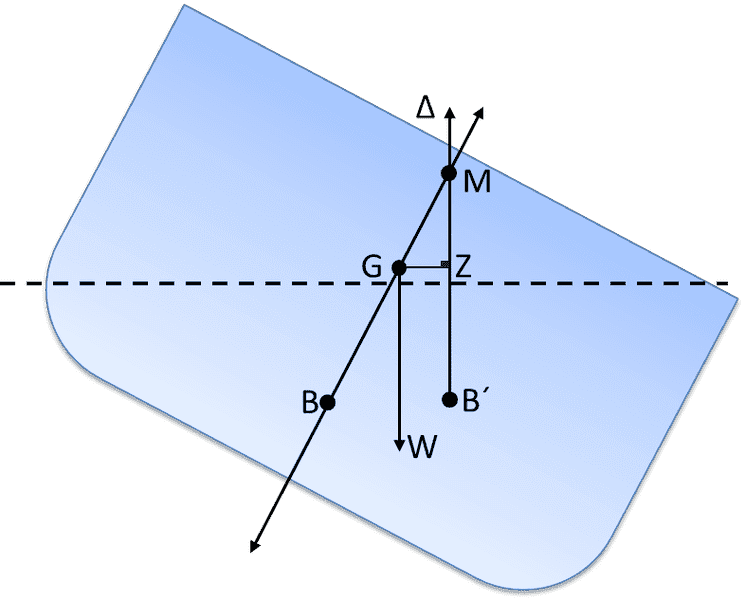Just like the weight of the vessel acts thru the point G, so does the buoyant force created by the displaced water act thru the point B and then B' after the vessel heels. The line of action of the buoyant force intersects the centerline of the vessel at a point M, also known as the metacenter. This point M is always located above B for floating vessels, and the distance BM = I / V.

For submerged vessels, there is no waterplane and hence no point M. A fully submerged vessel remains stable if and only if the center of gravity G remains below the center of buoyancy B.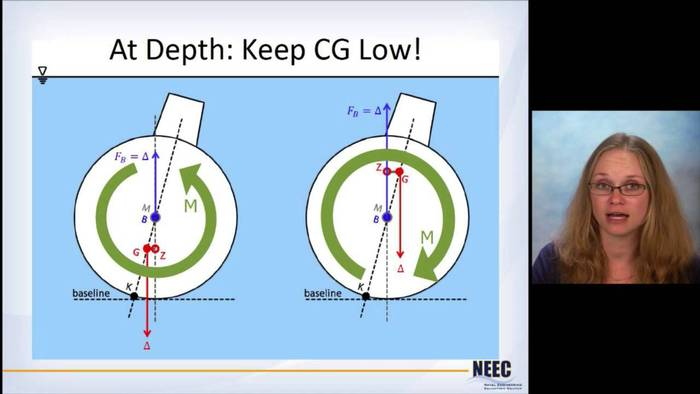When a floating vessel heels to one side, there is a slight shift in the location of the center of buoyancy to the side where the vessel is lower in the water, like this: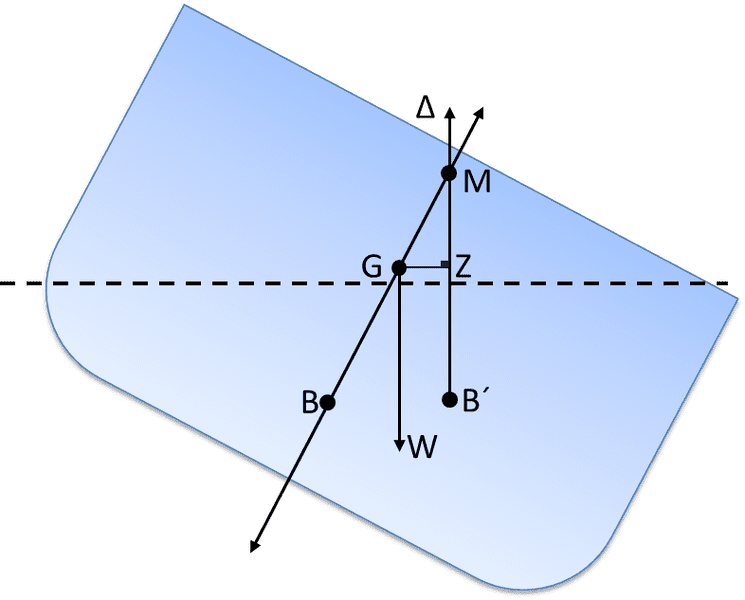Just like the weight of the vessel acts thru the point G, so does the buoyant force created by the displaced water act thru the point B and then B' after the vessel heels. The line of action of the buoyant force intersects the centerline of the vessel at a point M, also known as the metacenter. This point M is always located above B for floating vessels, and the distance BM = I / V.

For submerged vessels, there is no waterplane and hence no point M. A fully submerged vessel remains stable if and only if the center of gravity G remains below the center of buoyancy B.there is also occasion that the M will be below G (for floating body) , right ?it's unstable , then in thi case , where is the position of M and B ? M will still above B ?

there is also occasion that the M will be below G (for floating body) , right ?it's unstable , then in thi case , where is the position of M and B ? M will still above B ?
Yes. When G is above M, the metacentric height is negative and the vessel is unstable. M is still above B, however.

•foo9008
Yes. When G is above M, the metacentric height is negative and the vessel is unstable. M is still above B, however.
so, there are three arrangement only ? from the top top the bottom , 1st arrangement G , M , B , the arrangemnt is unstable
2nd arrangement , M , G, B, the arrangement is stable ,
3rd arrangement , M,B,G , the arrangement is stable

so, there are three arrangement only ? from the top top the bottom , 1st arrangement G , M , B , the arrangemnt is unstable
2nd arrangement , M , G, B, the arrangement is stable ,
3rd arrangement , M,B,G , the arrangement is stable
Anything with G below M is stable for floating bodies. For fully submerged bodies, there is no M, and G must be below B in order to be stable.

•foo9008
Hi, I was thinking if anyone knows the equation of finding BM, i.e. BM=I/V. But is this always true? or is it only for small angle of tilt?

Hi, I was thinking if anyone knows the equation of finding BM, i.e. BM=I/V. But is this always true? or is it only for small angle of tilt?
The metacentric height assesses the stability of a floating vessel only for small angles of heel, pretty much those angles where the size and shape of the waterplane are not affected to a great degree.

For assessing the stability of a vessel at larger angles of heel, the lateral separation of the lines of action of the buoyant force and the gravitational force acting on the vessel is calculated. This lateral separation is called the "righting lever" and is calculated for a series of angles starting at zero degrees and running through 90 degrees or greater as needed. The following diagram shows how B moves in relation to G as a vessel heels, and how this movement produces the righting lever which tends to move the vessel back to the upright position: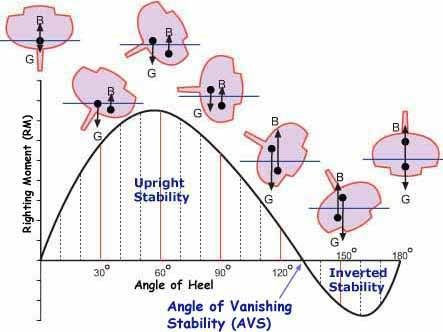Some vessels exhibit positive righting levers thru the full 360-degree range of heel. These vessels are called "self-righting", since they will not remain capsized if they are free to move.

In general, the calculation of the righting levers for a vessel is quite complicated and requires the use of special software designed for the purpose. For commercial vessels, the calculation of righting levers and the application of various stability criteria based on them are required in order to receive a certificate to operate.

The metacentric height assesses the stability of a floating vessel only for small angles of heel, pretty much those angles where the size and shape of the waterplane are not affected to a great degree.

For assessing the stability of a vessel at larger angles of heel, the lateral separation of the lines of action of the buoyant force and the gravitational force acting on the vessel is calculated. This lateral separation is called the "righting lever" and is calculated for a series of angles starting at zero degrees and running through 90 degrees or greater as needed. The following diagram shows how B moves in relation to G as a vessel heels, and how this movement produces the righting lever which tends to move the vessel back to the upright position:Some vessels exhibit positive righting levers thru the full 360-degree range of heel. These vessels are called "self-righting", since they will not remain capsized if they are free to move.

In general, the calculation of the righting levers for a vessel is quite complicated and requires the use of special software designed for the purpose. For commercial vessels, the calculation of righting levers and the application of various stability criteria based on them are required in order to receive a certificate to operate.
Hi, thanks for the explanation! It is very clear and useful.